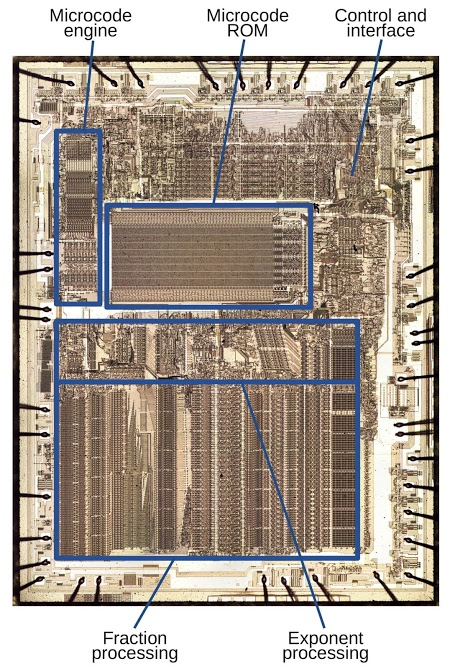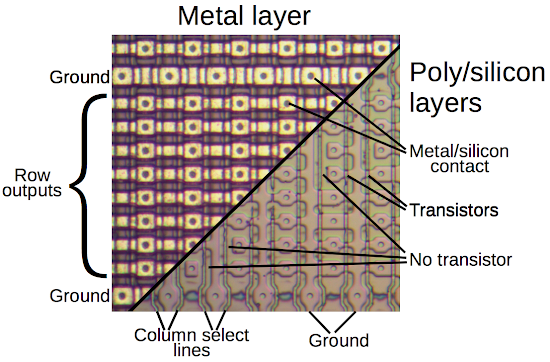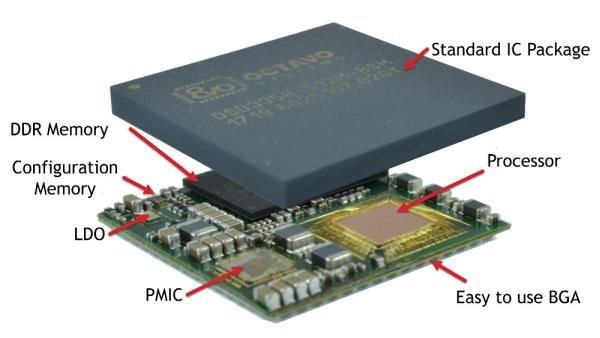# Two bits per transistor: high-density ROM in Intel’s 8087 floating point chip

The 8087 chip provided fast floating point arithmetic for the original IBM PC and became part of the x86 architecture used today. One unusual feature of the 8087 is it contained a multi-level ROM (Read-Only Memory) that stored two bits per transistor, twice as dense as a normal ROM. Instead of storing binary data, each cell in the 8087’s ROM stored one of four different values, which were then decoded into two bits. Because the 8087 required a large ROM for microcode1 and the chip was pushing the limits of how many transistors could fit on a chip, Intel used this special technique to make the ROM fit. In this article, I explain how Intel implemented this multi-level ROM.

Intel introduced the 8087 chip in 1980 to improve floating-point performance on the 8086 and 8088 processors. Since early microprocessors operated only on integers, arithmetic with floating point numbers was slow and transcendental operations such as trig or logarithms were even worse. Adding the 8087 co-processor chip to a system made floating point operations up to 100 times faster. The 8087’s architecture became part of later Intel processors, and the 8087’s instructions (although now obsolete) are still a part of today’s x86 desktop computers.

I opened up an 8087 chip and took die photos with a microscope yielding the composite photo below. The labels show the main functional blocks, based on my reverse engineering. (Click here for a larger image.) The die of the 8087 is complex, with 40,000 transistors.2 Internally, the 8087 uses 80-bit floating point numbers with a 64-bit fraction (also called significand or mantissa), a 15-bit exponent and a sign bit. (For a base-10 analogy, in the number 6.02×1023, 6.02 is the fraction and 23 is the exponent.) At the bottom of the die, “fraction processing” indicates the circuitry for the fraction: from left to right, this includes storage of constants, a 64-bit shifter, the 64-bit adder/subtracter, and the register stack. Above this is the circuitry to process the exponent.

READ  Micro camera module fits space-constrained devicesAn 8087 instruction required multiple steps, over 1000 in some cases. The 8087 used microcode to specify the low-level operations at each step: the shifts, adds, memory fetches, reads of constants, and so forth. You can think of microcode as a simple program, written in micro-instructions, where each micro-instruction generated control signals for the different components of the chip. In the die photo above, you can see the ROM that holds the 8087’s microcode program. The ROM takes up a large fraction of the chip, showing why the compact multi-level ROM was necessary. To the left of the ROM is the “engine” that ran the microcode program, essentially a simple CPU.

The 8087 operated as a co-processor with the 8086 processor. When the 8086 encountered a special floating point instruction, the processor ignored it and let the 8087 execute the instruction in parallel.3 I won’t explain in detail how the 8087 works internally, but as an overview, floating point operations were implemented using integer adds/subtracts and shifts. To add or subtract two floating point numbers, the 8087 shifted the numbers until the binary points (i.e. the decimal points but in binary) lined up, and then added or subtracted the fraction. Multiplication, division, and square root were performed through repeated shifts and adds or subtracts. Transcendental operations (tan, arctan, log, power) used CORDIC algorithms, which use shifts and adds of special constants, processing one bit at a time. The 8087 also dealt with many special cases: infinities, overflows, NaN (not a number), denormalized numbers, and several rounding modes. The microcode stored in ROM controlled all these operations.

READ  Withings GO activity tracker teardown

## Implementation of a ROM

The 8087 chip consists of a tiny silicon die, with regions of the silicon doped with impurities to give them the desired semiconductor properties. On top of the silicon, polysilicon (a special type of silicon) formed wires and transistors. Finally, a metal layer on top wired the circuitry together. In the photo below, the left side shows a small part of the chip as it appears under a microscope, magnifying the yellowish metal wiring. On the right, the metal has been removed with acid, revealing the polysilicon and silicon. When polysilicon crosses silicon, a transistor is formed. The pink regions are doped silicon, and the thin vertical lines are the polysilicon. The small circles are contacts between the silicon and metal layers, connecting them together.While there are many ways of building a ROM, a typical way is to have a grid of “cells,” with each cell holding a bit. Each cell can have a transistor for a 0 bit, or lack a transistor for a 1 bit. In the diagram above, you can see the grid of cells with transistors (where silicon is present under the polysilicon) and missing transistors (where there are gaps in the silicon). To read from the ROM, one column select line is energized (based on the address) to select the bits stored in that column, yielding one output bit from each row. You can see the vertical polysilicon column select lines and the horizontal metal row outputs in the diagram. The vertical doped silicon lines are connected to ground.

The schematic below (corresponding to a 4×4 ROM segment) shows how the ROM functions. Each cell either has a transistor (black) or no transistor (grayed-out). When a polysilicon column select line is energized, the transistors in that column turn on and pull the corresponding metal row outputs to ground. (For our purposes, an NMOS transistor is like a switch that is open if the input (gate) is 0 and closed if the input is 1.) The row lines output the data stored in the selected column.

READ  Brand New BiCMOS Flexible Transistor